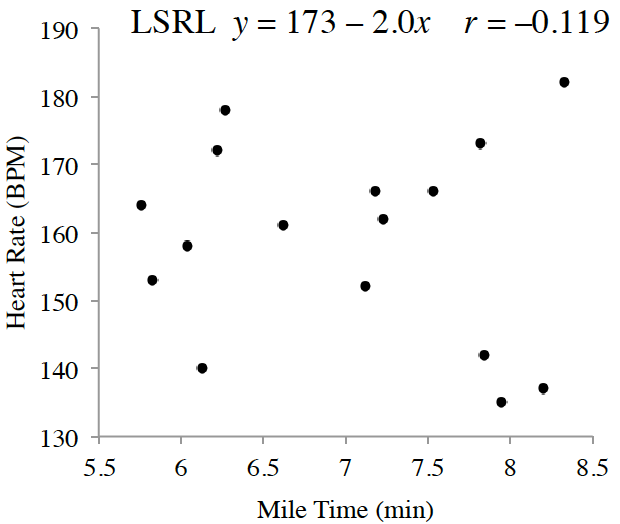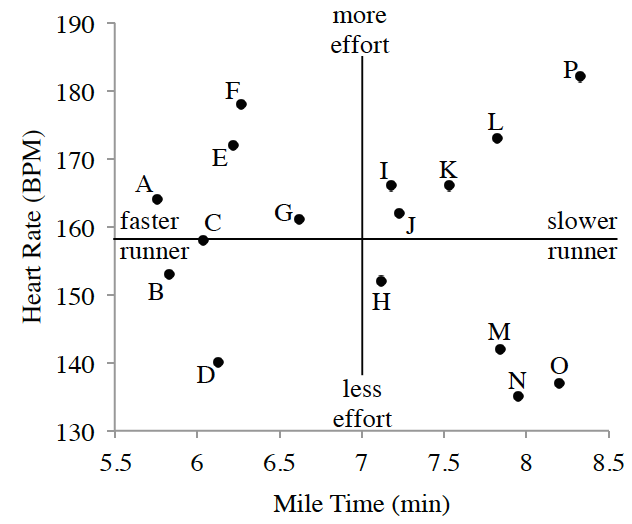### Home > CCAA8 > Chapter 9 Unit 10 > Lesson CCA: 9.4.2 > Problem9-109

9-109.

Coach Ron has $15$ athletes trying out for $12$ openings on the varsity basketball team. As part of the tryout he has each player run a mile and Ron records their time and heart rate as they finish. Coach Ron made a scatterplot as follows.Min. BPM A $5.76$ $164$ B $5.83$ $153$ C $6.04$ $158$ D $6.13$ $140$ E $6.22$ $172$ F $6.27$ $178$ G $6.62$ $161$ H $7.12$ $152$ I $7.18$ $166$ J $7.23$ $162$ K $7.53$ $166$ L $7.82$ $173$ M $7.84$ $142$ N $7.95$ $135$ O $8.2$ $137$ P $8.33$ $182$
1. Ron’s assistant Cheryl believes they can still find something useful in the results. She proposes that if heart rates could be considered the level of effort given, some athletes may stand out as more or less desirable for the team.

Cheryl divided the scatterplot into four quadrants and put the new labels on the axes. She also labeled each point. She drew the following scatterplot.

Describe the association to Coach Ron in detail.

2. Coach Cheryl believes that the farther a player is from the center, the more they help or hurt the team. Give specific advice to Coach Ron about players D, F, N, O and P.Is there any order to the data? Are there any outliers?

Example: D- Ran a fast mile but seemed to be giving little effort. This athlete might already be in outstanding physical condition or have an attitude problem.

Give Coach Ron similar advice about player F, N, O, and P.